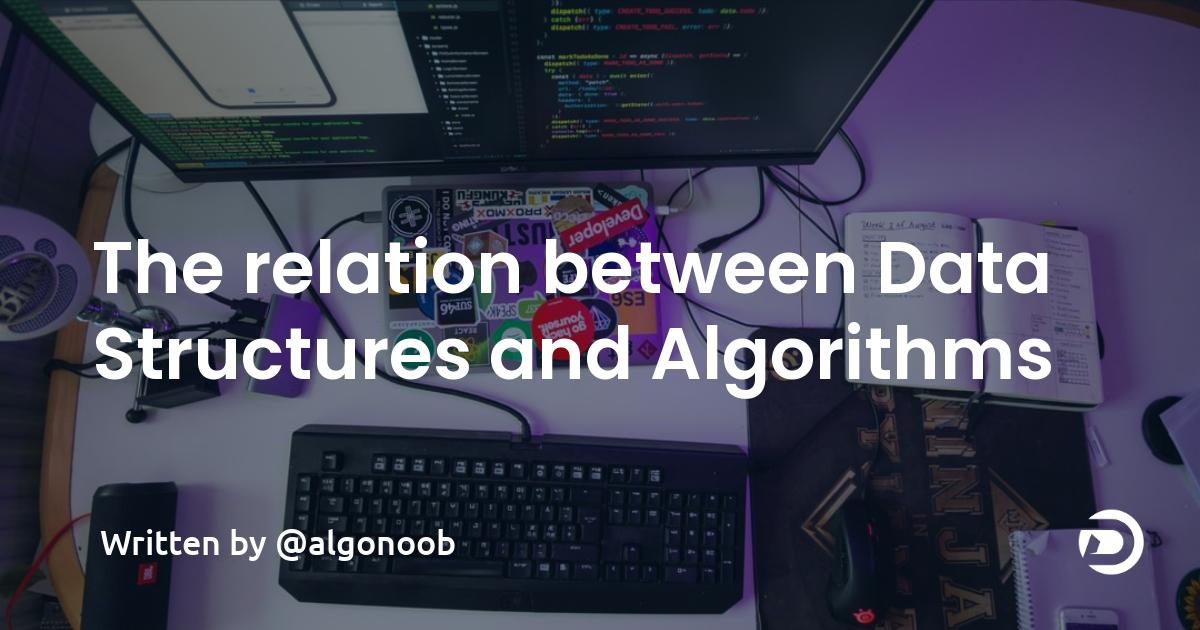# The relation between Data Structures and AlgorithmsCreated November 13, 2021

In this article we're gonna talk about the association between two very important components of programming, Data Structures and Algorithms.

## What are Data Structures?

Data Structures can be simply defined as the way of storing data in an organized way to make our work easier. Data Structures are important as they reduce the complexity of the process that is being performed using it and it considerably reduces the time spent on doing the procedure. The key idea is to use the most ideal data structure there is for doing a particular task.

## What are Algorithms?

Algorithms are simply the "process" that we were talking about in the previous definition. It can be defined as the set of steps that can be used to solve a certain problem. There can be many different ways of solving a problem. Ideally, we choose the most optimal algorithm there can be to solve a problem so as to solve it in the least time.

## The relation

To make an Algorithm optimal and least time taking, we make use of Data Structures in Algorithms. They considerably reduce the time complexity of the algorithm.

## An Example

Suppose that you've been assigned "n" random integers. You have to sort them in ascending order of their values. How would you go about it?

### The most basic solution

The first solution that might've crossed your mind maybe storing all the numbers in an array and then applying some sorting algorithm on it like bubble sort, selection sort, merge sort, etc.

If you apply bubble sort on it, the worst case time complexity of the whole process would be O(n²). In case of Mergesort, the worst case time complexity would be O(n*log(n)).